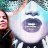# Need Stochastic indicator with multiple lengths

W

#### wsjiii

##### New member
Hello,

I'm trying to create a lower study (StochasticFull) with just the FullK input (no FullD) but at multiple periods. So instead of just one FullK stochastic showing at say 14 period, I want to show multiple FullK's, for example 7, 14 and 21, but all on the same study. I am having a heck of a time though. Any suggestions?#### horserider

##### Well-known member
VIP
Would need to input your KPeriod lengths. Kperiod, KPeriod2, KPeriod3
Replicate the KPeriod calculations for each KPeriod length.
Plot each calculation.

Do not peek. Code is below if you cannot figure it out.

Code:
``````# Three different lengths of StochasticCrossover FullK
# Horserider 1/12/2020 requested by wsjiii

declare lower;

input over_bought = 80;
input over_sold = 20;
input KPeriod = 7;
input KPeriod2 = 14;
input KPeriod3 = 21;
input priceH = high;
input priceL = low;
input priceC = close;
input slowing_period = 3;
input averageType = AverageType.SIMPLE;

# First K period
def lowest_k = Lowest(priceL, KPeriod);
def c1 = priceC - lowest_k;
def c2 = Highest(priceH, KPeriod) - lowest_k;
def FastK = if c2 != 0 then c1 / c2 * 100 else 0;

# Second K period
def lowest_k2 = Lowest(priceL, KPeriod2);
def c12 = priceC - lowest_k2;
def c22 = Highest(priceH, KPeriod2) - lowest_k2;
def FastK2 = if c22 != 0 then c12 / c22 * 100 else 0;

# Third K period
def lowest_k3 = Lowest(priceL, KPeriod3);
def c13 = priceC - lowest_k3;
def c23 = Highest(priceH, KPeriod3) - lowest_k3;
def FastK3 = if c23 != 0 then c13 / c23 * 100 else 0;

# Plots
plot FullK = MovingAverage(averageType, FastK, slowing_period);
plot FullK2 = MovingAverage(averageType, FastK2, slowing_period);
plot FullK3 = MovingAverage(averageType, FastK3, slowing_period);

plot OverBought = over_bought;
plot OverSold = over_sold;

FullK.SetDefaultColor(GetColor(2));
FullK2.SetDefaultColor(GetColor(3));
FullK3.SetDefaultColor(GetColor(4));
OverBought.SetDefaultColor(GetColor(1));
OverSold.SetDefaultColor(GetColor(1));``````

Last edited by a moderator:
W

#### wsjiii

##### New member
Thanks a lot for the help. It seems I was over-complicating things...Need % percentage change in Stochastic Questions 2